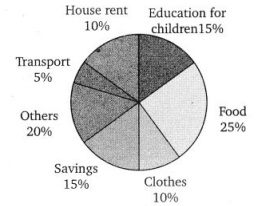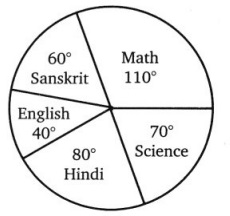# Grade 8 Data Handling Worksheets

## Grade 8 Maths Data Handling Multiple Choice Questions (MCQs)

Frequency Distribution of Daily Income of 550 workers of a factory is given below. Study the following frequency distribution table and answer the questions from Q1 – Q10.1. What is the size of class intervals?
(a) 24
(b) 25
(c) 26
(d) 15

2. Which class has the highest frequency?
(a) 200 – 225
(b) 300 – 325
(c) 175 – 200
(d) 150 – 175

3. Which class has the lowest frequency?
(a) 100 – 125
(b) 300 – 325
(c) 175 – 200
(d) 150 – 175

4. What is the upper limit of the class interval 250 – 275?
(a) 250
(b) 275
(c) 25
(d) 525

5. Which two classes have the same frequency?
(a) III & VI
(b) I & II
(c) II & V
(d) V & VI

6. What is the range of all the class interval?
(a) 250
(b) 275
(c) 225
(d) 525

7. What is the lower limit of the class interval 250 – 275?
(a) 250
(b) 275
(c) 25
(d) 525

8. What is the total number of workers having daily income less than 250?
(a) 300
(b) 445
(c) 305
(d) 550

9. What is the total number of workers having daily income more than 200?
(a) 300
(b) 445
(c) 305
(d) 550

10. What is the total number of workers having daily income between 150-250?
(a) 300
(b) 445
(c) 375
(d) 550

Cards marked with numbers 1 to 25 are placed in the box and mixed thoroughly. One card is drawn at random from the box. Answer the following questions (Q11 – Q20):

11. What is the probability of getting number 5?
(a) 1
(b) 0
(c)$\frac{1}{25}$
(d)$\frac{1}{5}$

12. What is the probability of getting number less than 11?
(a) 1
(b) 0
(c)$\frac{1}{5}$
(d)$\frac{2}{5}$

13. What is the probability of getting number greater than 25?
(a) 1
(b) 0
(c)$\frac{1}{5}$
(d)$\frac{2}{5}$

14. What is the probability of getting a multiple of 5?
(a) 1
(b) 0
(c)$\frac{1}{25}$
(d)$\frac{1}{5}$

15. What is the probability of getting an even number?
(a) 1
(b) 0
(c)$\frac{12}{25}$
(d)$\frac{13}{25}$

16. What is the probability of getting an odd number?
(a) 1
(b) 0
(c)$\frac{12}{25}$
(d)$\frac{13}{25}$

17. What is the probability of getting a prime number?
(a)$\frac{8}{25}$
(b)$\frac{9}{25}$
(c)$\frac{12}{25}$
(d)$\frac{13}{25}$

18. What is the probability of getting a number divisible by 3?
(a)$\frac{8}{25}$
(b)$\frac{9}{25}$
(c)$\frac{12}{25}$
(d)$\frac{13}{25}$

19. What is the probability of getting a number divisible by 4?
(a)$\frac{8}{25}$
(b)$\frac{9}{25}$
(c)$\frac{6}{25}$
(d)$\frac{3}{25}$

20. What is the probability of getting a number divisible by 7?
(a)$\frac{8}{25}$
(b)$\frac{9}{25}$
(c)$\frac{6}{25}$
(d)$\frac{3}{25}$

### Class 8 Maths Data Handling Fill In The Blanks

1. The information collected in terms of number is called ………………… .
2. ……………….. is the difference between the maximum and minimum observation in the data.
3. Data available in an unorganized form is called …………………… data.
4. A pie chart is used to compare ………………….. to whole.
5. When the observations is large, the observations are usually organized in groups of equal width called ………………… .
6. The class size of the interval 40 – 45 is ………………… .
7. In a histogram …………………… are drawn with width equal to a class interval without leaving, any gap in between.
8. The probability of an event cannot be ………………… than one.

### Class 8 Maths Data Handling True(T) Or False(F)

1. Probability of an event may be less than zero.
2. In a single throw of dice probability of getting a number less than 7 is zero.
3. In a pie chart a whole circle is divided into sectors.
4. The sum of central angles of a sector in a pie chart is 360°.
5. In a pie chart two or more central angles can be equal.
6. The probability of getting a prime number is the same as that of a composite number in a throw of dice.
7. The probability of getting a prime number is the same as that of a composite number is the same as that of getting an odd number.
8. The probability of getting number 6 in a throw of dice is$\frac{1}{6}$. Similarly the probability of getting number 3 is$\frac{1}{3}$.

Adjoining pie-chart gives the expenditure (in % age) on various items and savings of a family during a month. Study the given pie-chart and answer the questions from (1 – 10):1. On which item the expenditure was maximum?
2. On which item the expenditure was minimum?
3. Expenditure on which item is equal to total savings of the family?
4. Expenditure on which item is equal to the House Rent?

If the monthly savings of the family is ₹ 3000:

5. What is the monthly income of the family?
6. What is the monthly expenditure on cloths?
7. What is the monthly expenditure on education for children?
8. What is the monthly expenditure on others?
9. What is the monthly expenditure on Transport?
10. What is the monthly expenditure on Food?

The following pie chart shows the marks obtained by a student of class VIII in six subjects:

If the total marks are 180 than, what is the difference of marks obtained in Maths and Science?